# Ultra-Low Resistance Surface Mount Current Shunts

### 要約

Ultra-low resistance surface mount current-sense resistors seem like the ideal package. The physical design, a strap of metal, seems ideal, offering the lowest inductance possible. Too often these resistors need some kind of resistor capacitor filter to limit the switching noise spikes. The problem is that the time constant for the shunt is the package inductance divided by the circuit resistance. Consequently, the lower the shunt resistance, the longer the decay times.

Ultra-low resistance surface mount current-sense resistors seem like the ideal package. The physical design, a strap of metal, seems ideal, offering the lowest inductance possible. Too often these resistors need some kind of resistor capacitor filter to limit the switching noise spikes. The problem is that the time constant for the shunt is the package inductance divided by the circuit resistance. Consequently, the lower the shunt resistance, the longer the decay times.

Most ultra-low resistance surface mount components model like a piece of wire. The ideal circuit model is a resistor. Many engineers deploy a four terminal Kelvin sensing method to reduce errors in the ground plane. This does nothing for errors in the sense resistor itself. A 1-watt, 0.005Ω surface mount resistor can have as much as 5nH of package inductance. When relying on the resistive element in a current measuring application, the circuit will have a limited frequency that can be defined as the point that the inductive reactance equals the shunt resistance, or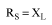WhereIn the case shown in Figure 1, the upper usable frequency limit is 160kHz! The time constant for the RL network is L/R or 1uS. For lower resistance values in the same package the problem only gets worse.Figure 1.

This problem can be corrected with the RC circuit as shown in Figure 2. For a current-sense application we desire I(jw) × Rs = V2. The circuit in Figure 2 can be made to give this result by making the pole in the R1, C1 network cancel the zero in the shunt resistor.Figure 2.

Since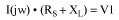andit follows that by substituting V1 in the second equation we haveSince we desirewe can modify the above expression asBy simplifying we find thatand by cancelling the two radicals we have the desired result. From here we makewhere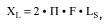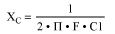and chooseWith simplification we find thatorwhich simplifies to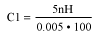so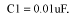This model assumes the shunt is driven from a current source (high impedance) such as an inductor. It must be noted that the network in Figure 2 does not provide high-frequency filtering, because the response is flat. A second pole must be added for high-frequency rolloff. Driving the shunt with low impedance, such as a MOSFET source, introduces a pole at V1. This pole occurs when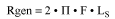orIf desired, this pole can be cancelled by inserting a zero with R2. If further accuracy is required you might try using potentiometers for R1 and R2 with your best guess for C1.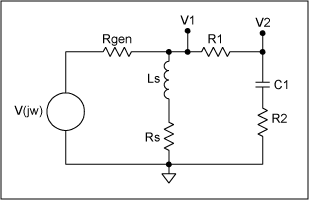Figure 3.

Measuring these ultra-low resistance components can tax the most expensive inductance bridges and network analyzers to the limit of their capacity. Using high-frequency sweeps and maximum stimulus levels can help to bring these measurements up out of the noise floor. But circuit layouts, such as ground planes, can change the measured results.

In conclusion, it seems that the ideal current-sense resistor does not yet exist. As power-supply output currents continue to rise, these shunt resistors will probably be entering the sub-milliohm level very soon. Along with increased operating frequency, caution for these second and third order effects is good advice.